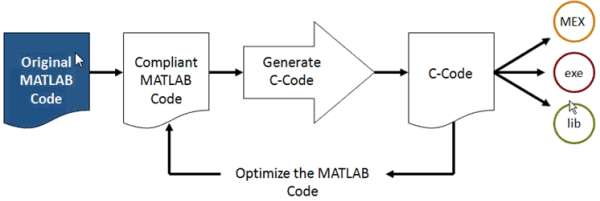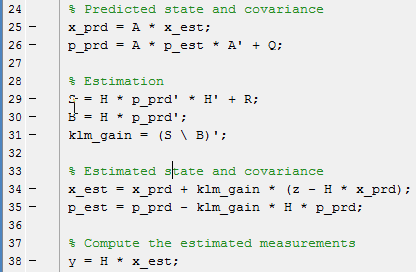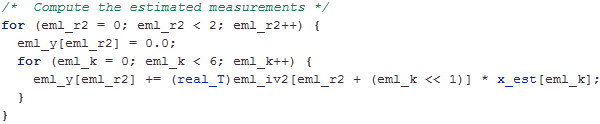# Kalman Filter – From MATLAB to Embedded C CodeBy Guy Rouleau

In a previous post, I introduced the Embedded MATLAB Function block. This block gives the possibility to write an algorithm in MATLAB code, include it in a Simulink model, and generate embedded C code using Real-Time Workshop.

When seeing this, some users, especially from the signal processing and communication domain asked: Do I really need Simulink to generate embedded C code from a MATLAB function?

To answer this question, I would like to highlight a webinar and a submission on the MATLAB Central File Exchange from my colleague Houman Zarrinkoub:

Webinar: MATLAB to C Code Generation with Embedded MATLAB

File Exchange Submission: Kalman filtering demo in MATLAB with automatic MATLAB-to-C code generation

Using Embedded MATLAB, you can generate C-code and test your algorithm on the real hardware with only a few clicks. It significantly reduces the turnaround time of design iterations.For the Kalman filter in Houman's submission, the equations are implemented in MATLAB as you would see them in a book:Then using emlc, the algorithm is converted to C code. For example, the last line of the above code is turned into:So now I recommend that you get yourself a drink, sit down and watch Houman's webinar!